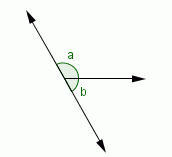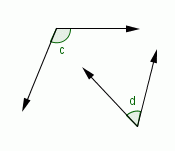Email us to get an instant 20% discount on highly effective K-12 Math & English kwizNET Programs!

#### Online Quiz (WorksheetABCD)

Questions Per Quiz = 2 4 6 8 10

### MEAP Preparation - Grade 7 Mathematics4.7 Supplementary Angles

 Two angles are said to be supplementary if the sum of their measures is 180�. Adjacent and nonadjacent supplementary angles are shown below: m�a + m�b = 1800 m�c + m�d = 1800Example: If the measure of an angle is 20�, what is the measure of its supplement? Solution: The measure of the supplement = 180� - 20� = 160� Directions: For the following questions, find the measure of the supplement of the angle given, draw the figure and enter only the number in the boxes. For example, the supplement of the angle 70 degrees is 110.
 Q 1: If the measure of an angle is 120 degrees, what is the measure of its supplement?Answer: Q 2: If the measure of an angle is 72 degrees, what is the measure of its supplement?Answer: Q 3: If the measure of an angle is 90 degrees, what is the measure of its supplement?Answer: Q 4: If the measure of an angle is 10 degrees, what is the measure of its supplement?Answer: Q 5: If the measure of an angle is 145 degrees, what is the measure of its supplement?Answer: Q 6: If the measure of an angle is 168 degrees, what is the measure of its supplement?Answer: Q 7: If the measure of an angle is 70 degrees, what is the measure of its supplement?Answer: Q 8: If the measure of an angle is 150 degrees, what is the measure of its supplement?Answer: Question 9: This question is available to subscribers only! Question 10: This question is available to subscribers only!